Скачать презентацию Integrating Math Units and Proof Checking for Specification

• Количество слайдов: 38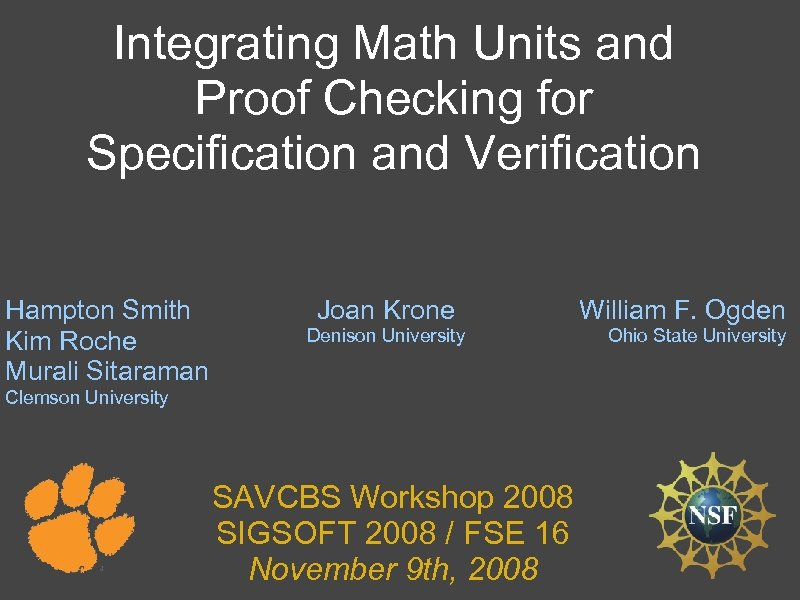Integrating Math Units and Proof Checking for Specification and Verification Hampton Smith Kim Roche Murali Sitaraman Joan Krone Denison University Clemson University SAVCBS Workshop 2008 SIGSOFT 2008 / FSE 16 November 9 th, 2008 William F. Ogden Ohio State University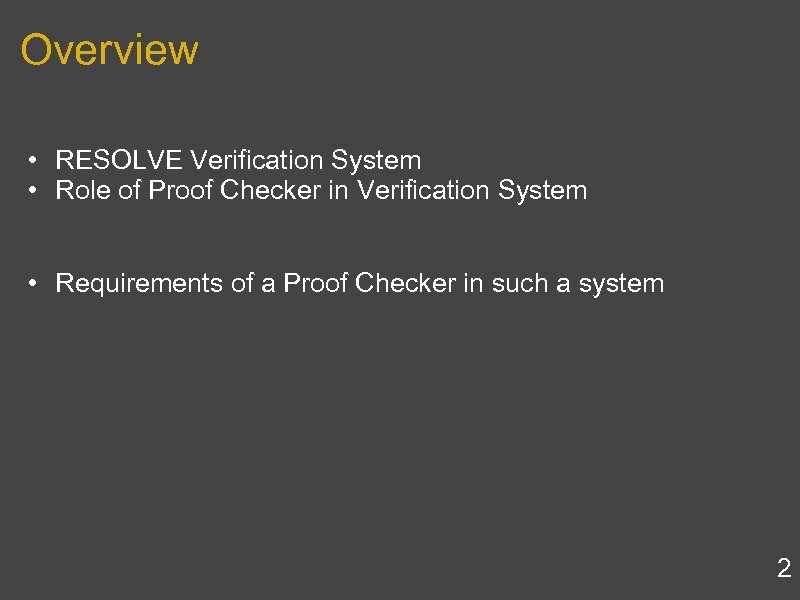Overview • RESOLVE Verification System • Role of Proof Checker in Verification System • Requirements of a Proof Checker in such a system 2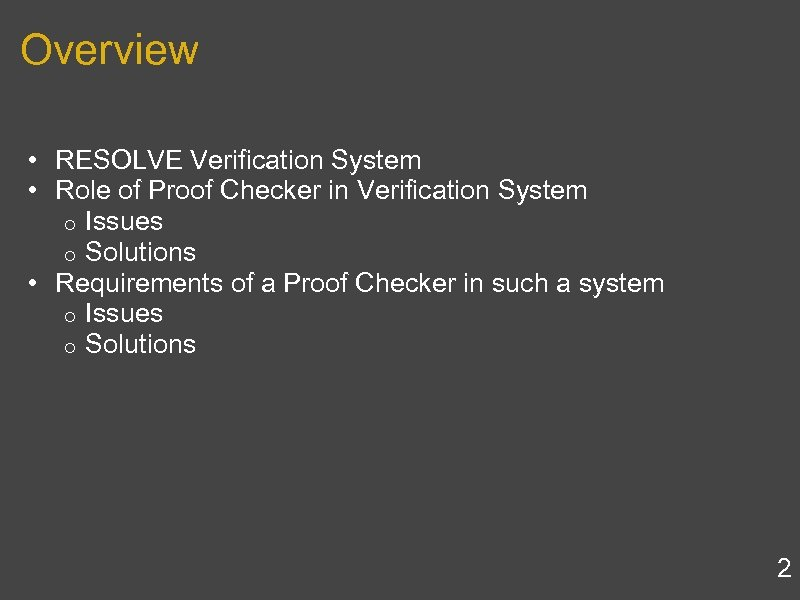Overview • RESOLVE Verification System • Role of Proof Checker in Verification System o Issues o Solutions • Requirements of a Proof Checker in such a system o Issues o Solutions 2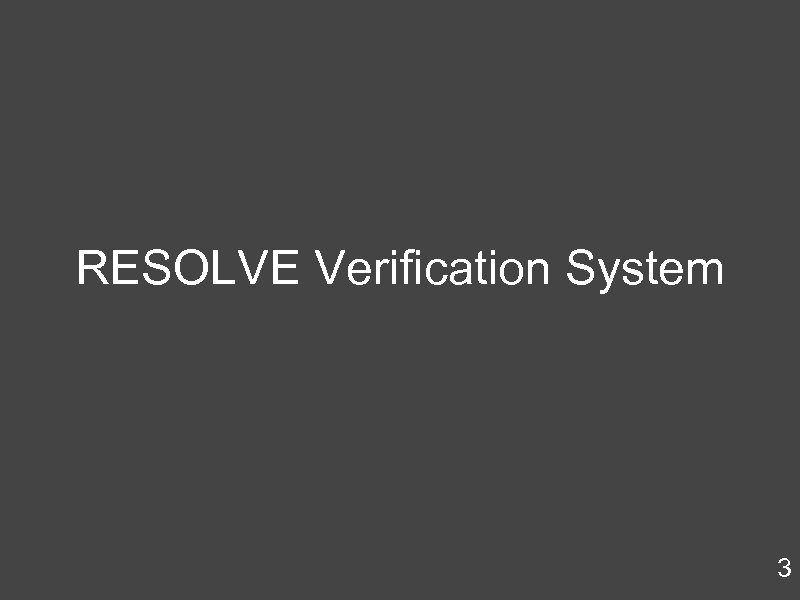RESOLVE Verification System 3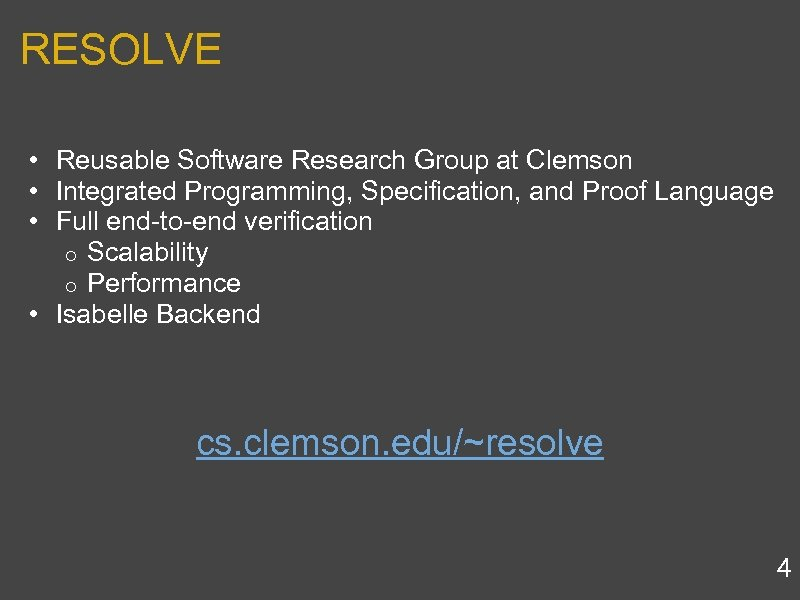RESOLVE • Reusable Software Research Group at Clemson • Integrated Programming, Specification, and Proof Language • Full end-to-end verification o Scalability o Performance • Isabelle Backend cs. clemson. edu/~resolve 4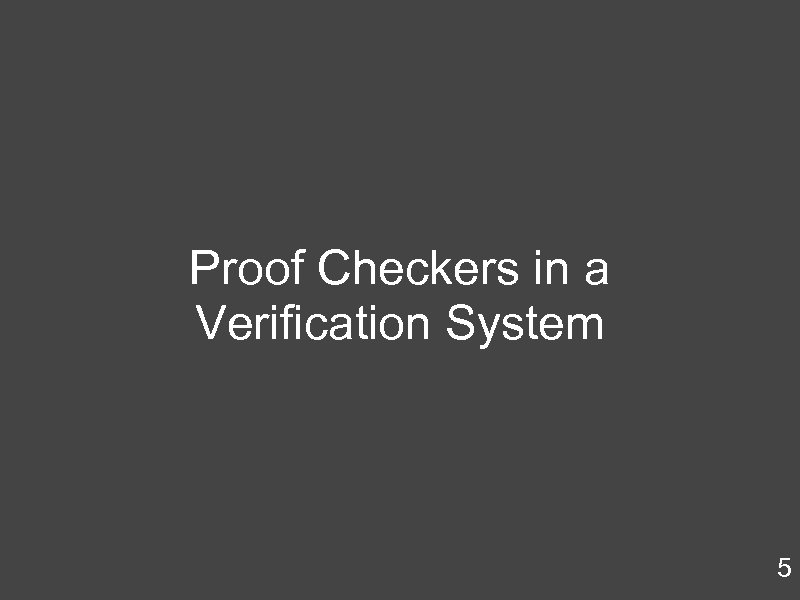Proof Checkers in a Verification System 5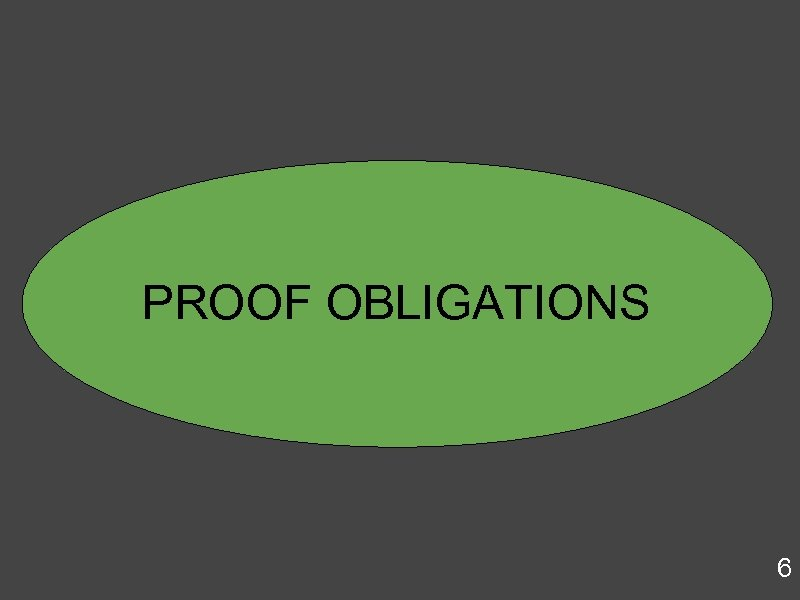PROOF OBLIGATIONS 6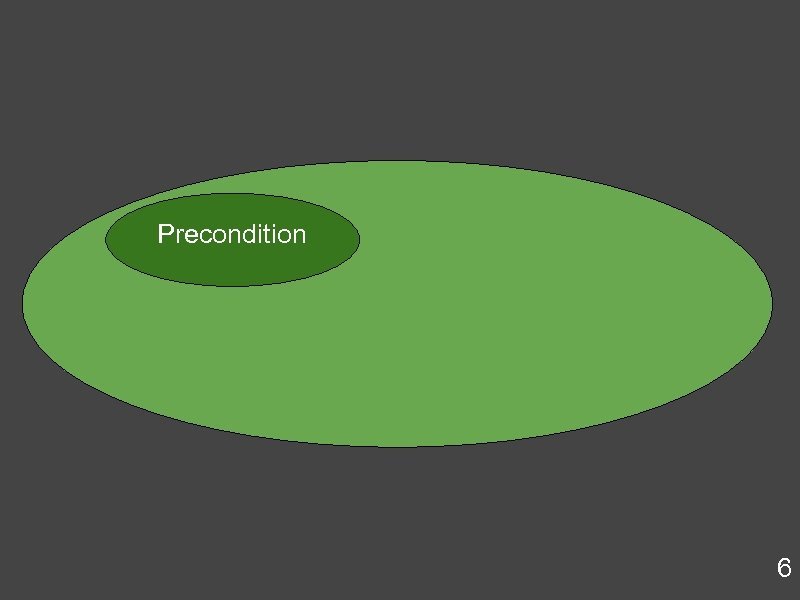Precondition 6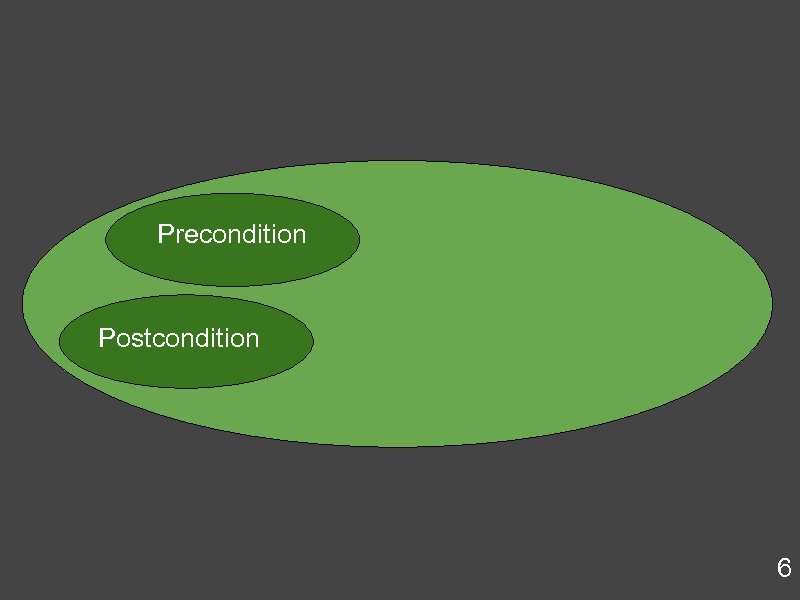Precondition Postcondition 6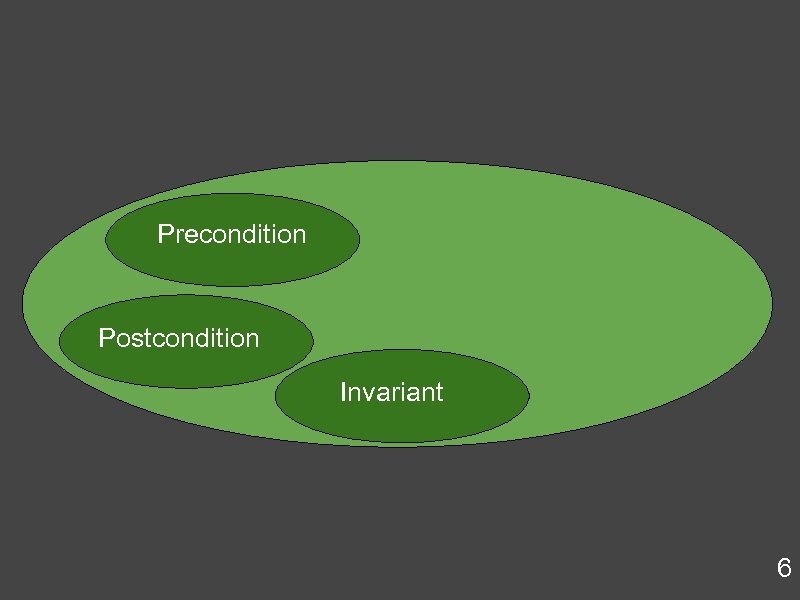Precondition Postcondition Invariant 6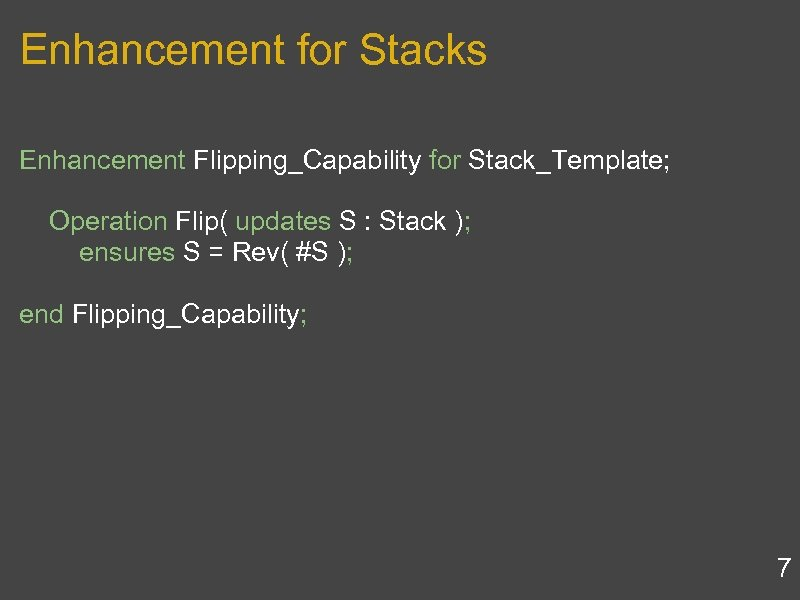Enhancement for Stacks Enhancement Flipping_Capability for Stack_Template; Operation Flip( updates S : Stack ); ensures S = Rev( #S ); end Flipping_Capability; 7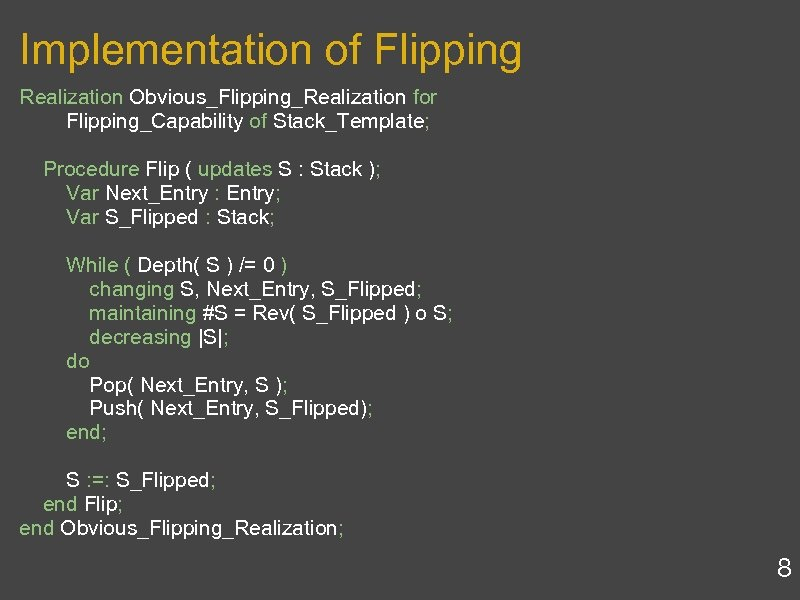Implementation of Flipping Realization Obvious_Flipping_Realization for Flipping_Capability of Stack_Template; Procedure Flip ( updates S : Stack ); Var Next_Entry : Entry; Var S_Flipped : Stack; While ( Depth( S ) /= 0 ) changing S, Next_Entry, S_Flipped; maintaining #S = Rev( S_Flipped ) o S; decreasing |S|; do Pop( Next_Entry, S ); Push( Next_Entry, S_Flipped); end; S : =: S_Flipped; end Flip; end Obvious_Flipping_Realization; 8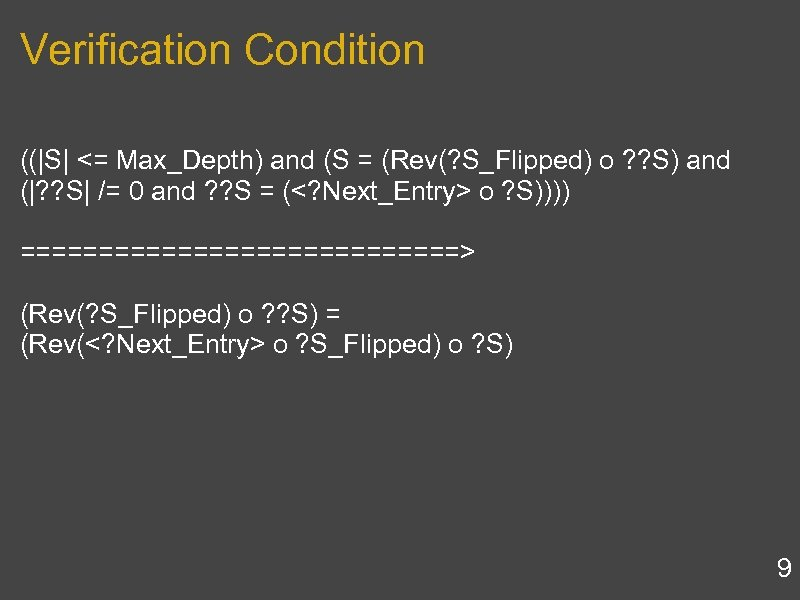Verification Condition ((|S| <= Max_Depth) and (S = (Rev(? S_Flipped) o ? ? S) and (|? ? S| /= 0 and ? ? S = ( o ? S)))) ==============> (Rev(? S_Flipped) o ? ? S) = (Rev( o ? S_Flipped) o ? S) 9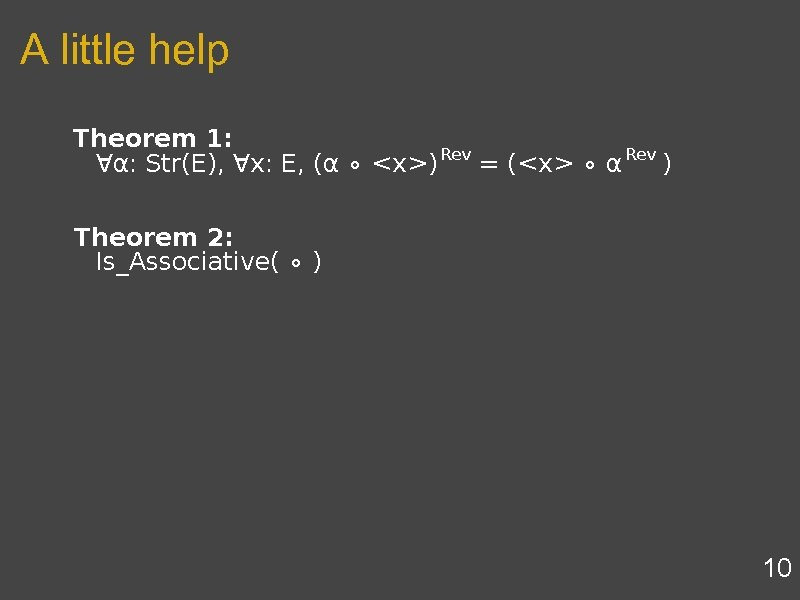A little help 10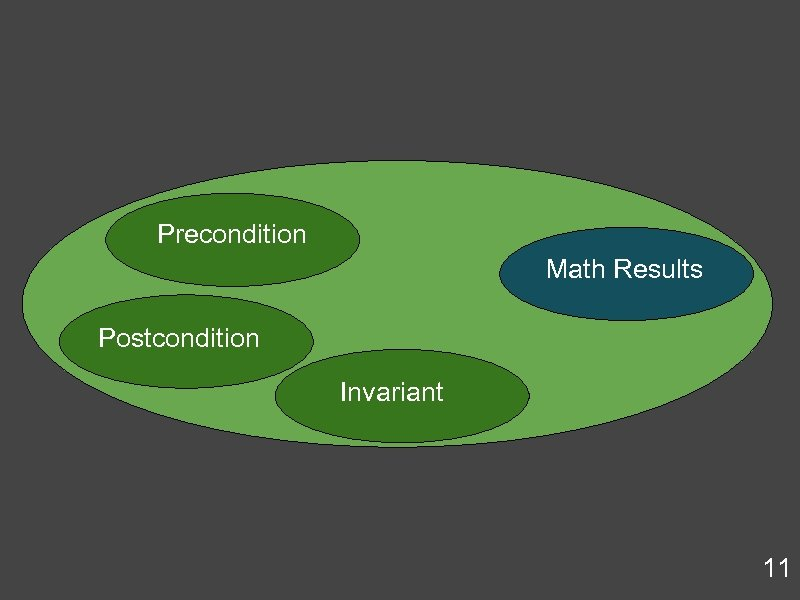Precondition Math Results Postcondition Invariant 11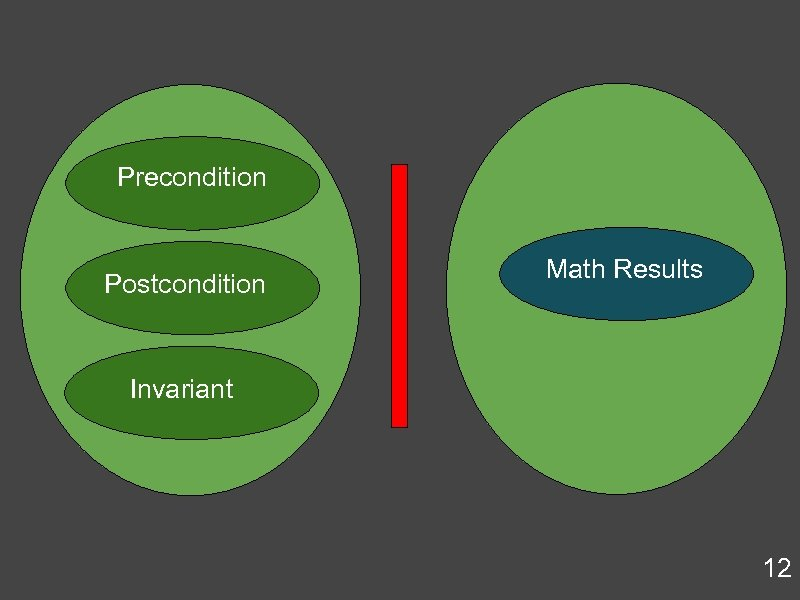Precondition Postcondition Math Results Invariant 12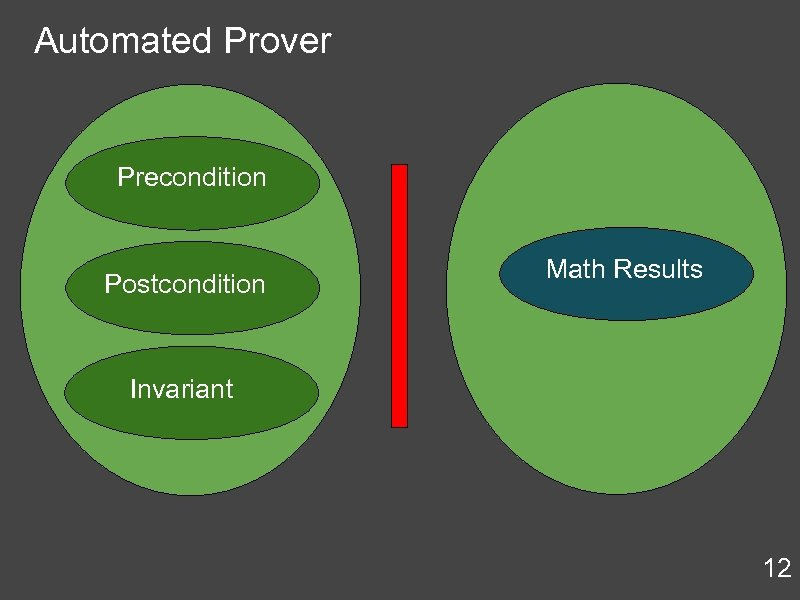Automated Prover Precondition Postcondition Math Results Invariant 12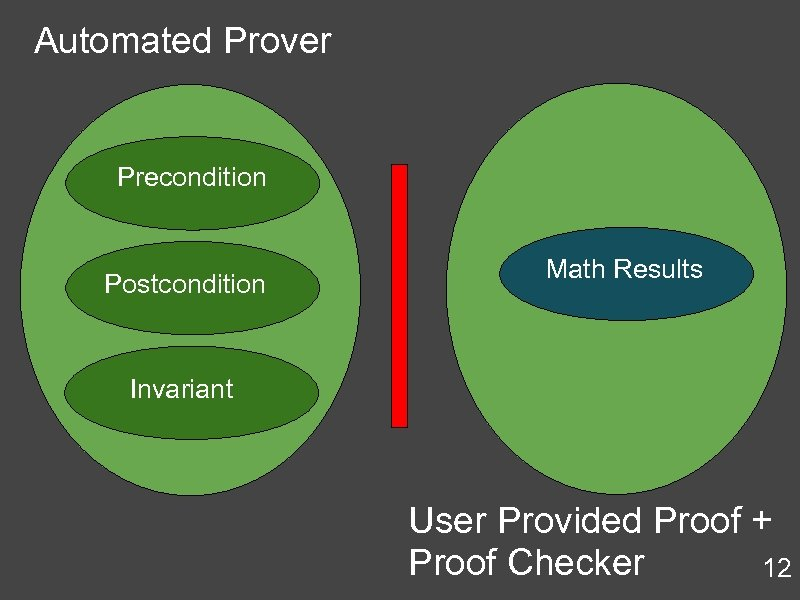Automated Prover Precondition Postcondition Math Results Invariant User Provided Proof + Proof Checker 12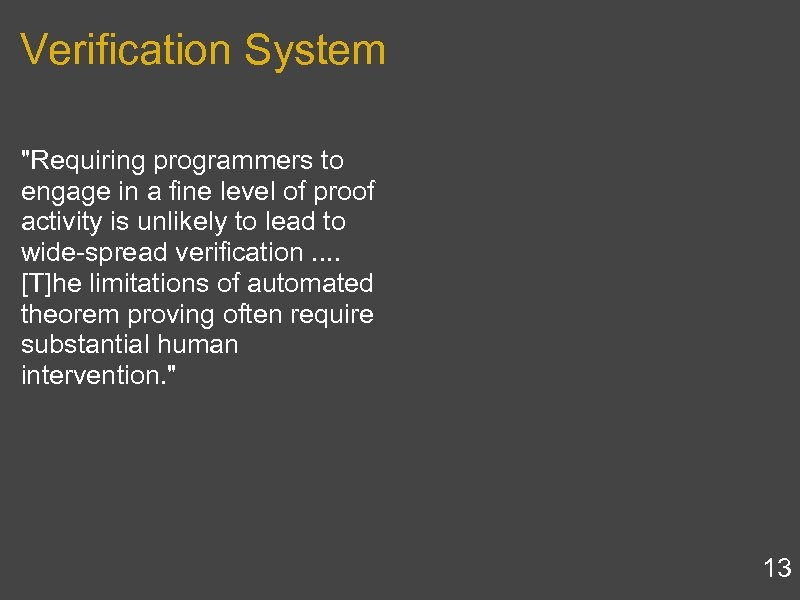Verification System "Requiring programmers to engage in a fine level of proof activity is unlikely to lead to wide-spread verification. . [T]he limitations of automated theorem proving often require substantial human intervention. " 13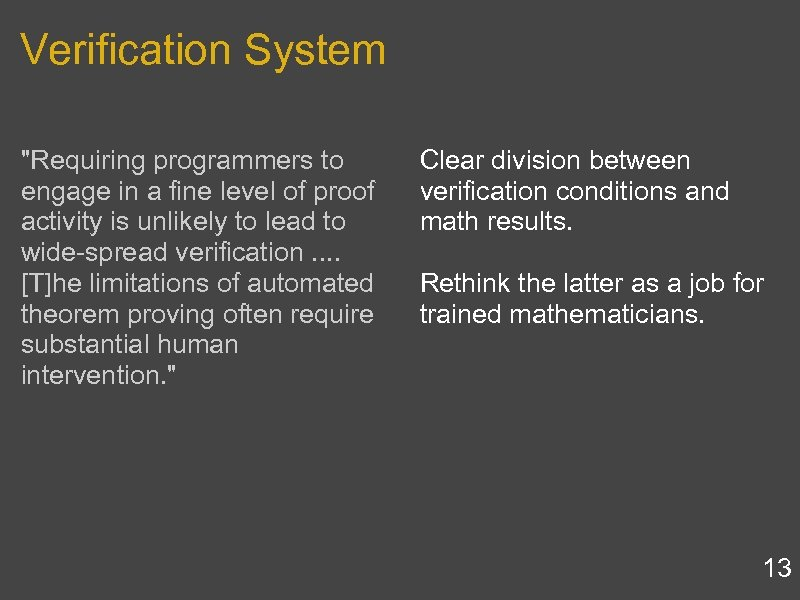Verification System "Requiring programmers to engage in a fine level of proof activity is unlikely to lead to wide-spread verification. . [T]he limitations of automated theorem proving often require substantial human intervention. " Clear division between verification conditions and math results. Rethink the latter as a job for trained mathematicians. 13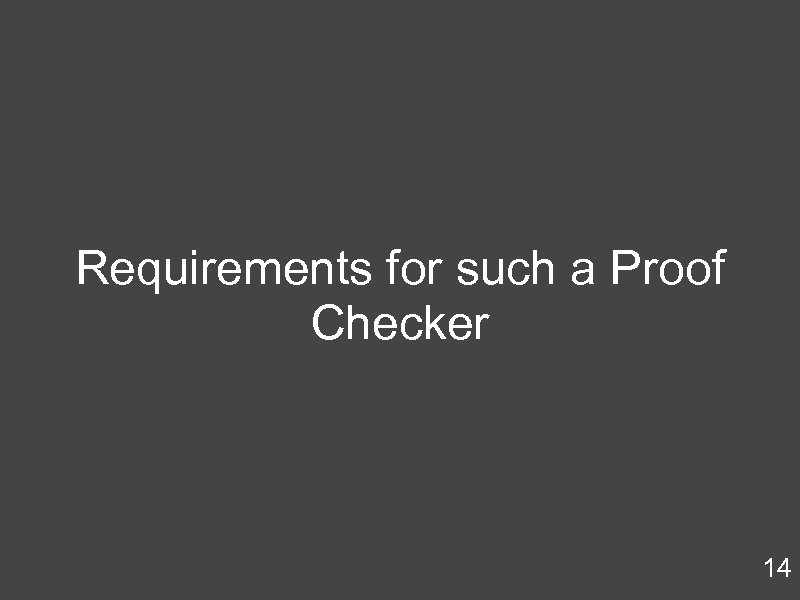Requirements for such a Proof Checker 14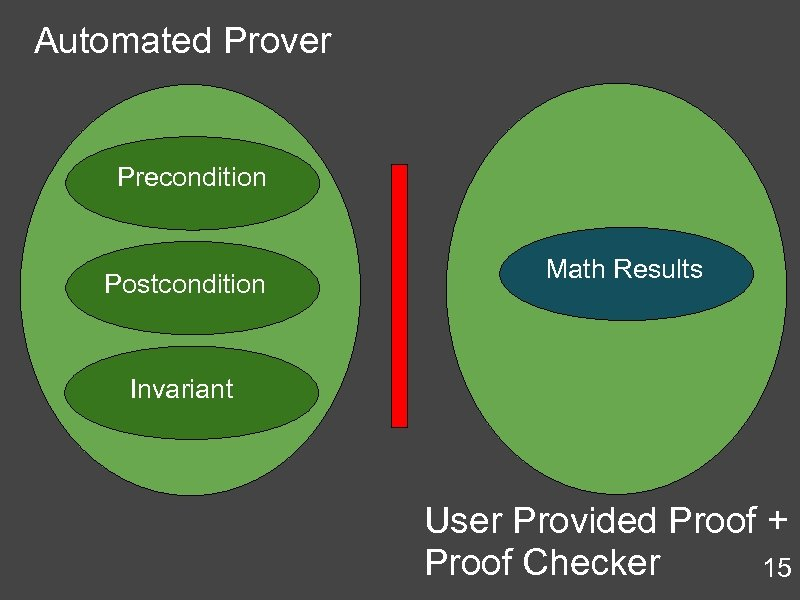Automated Prover Precondition Postcondition Math Results Invariant User Provided Proof + Proof Checker 15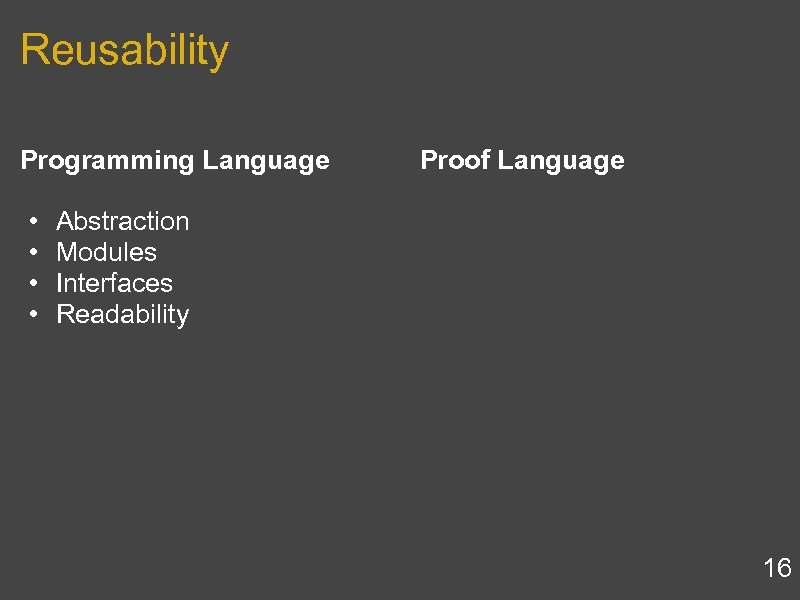Reusability Programming Language • Abstraction • Modules • Interfaces • Readability Proof Language 16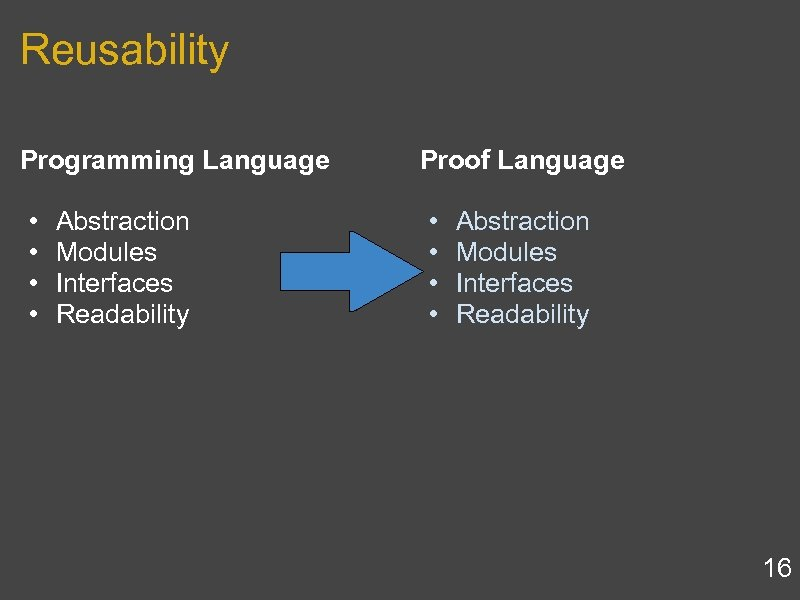Reusability Programming Language • Abstraction • Modules • Interfaces • Readability Proof Language • Abstraction • Modules • Interfaces • Readability 16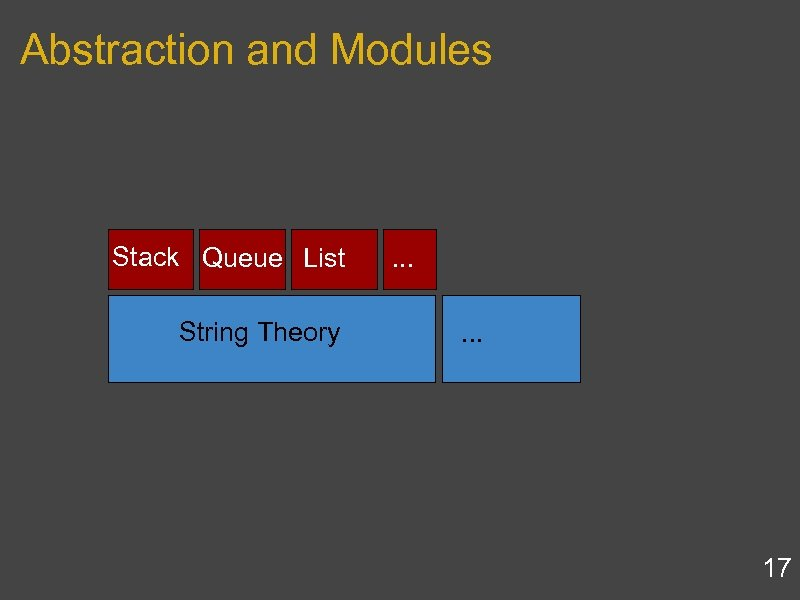Abstraction and Modules Stack Queue List String Theory . . . 17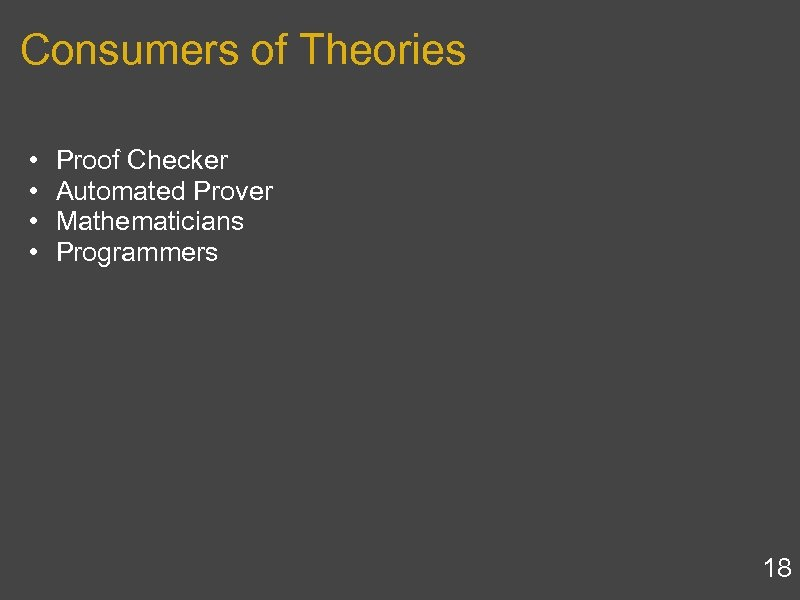Consumers of Theories • • Proof Checker Automated Prover Mathematicians Programmers 18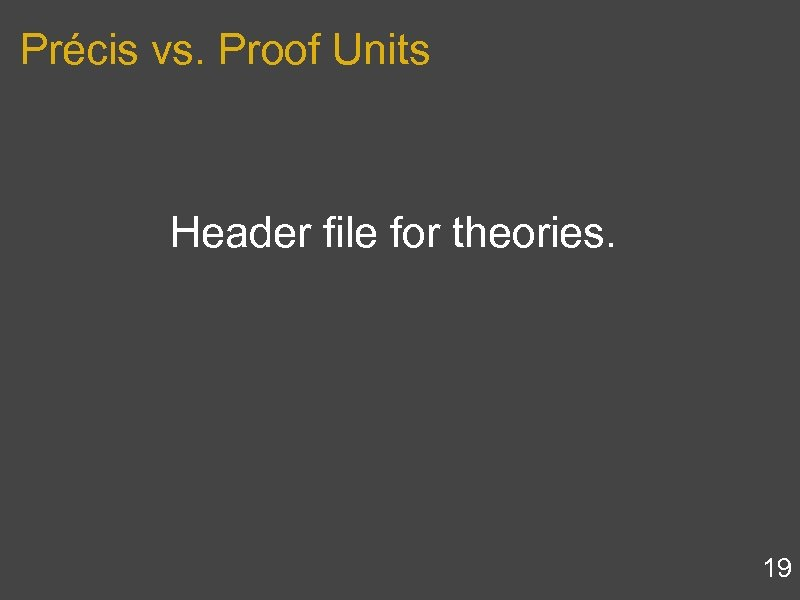Précis vs. Proof Units Header file for theories. 19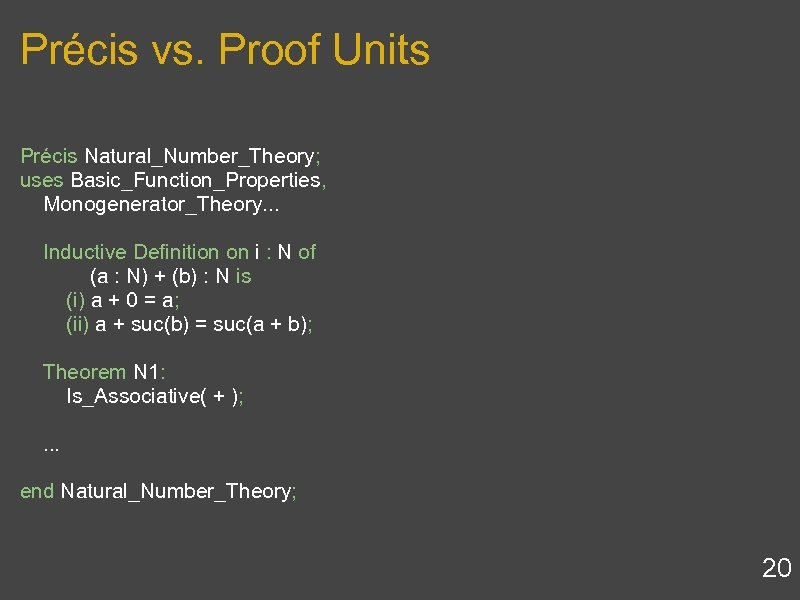Précis vs. Proof Units Précis Natural_Number_Theory; uses Basic_Function_Properties, Monogenerator_Theory. . . Inductive Definition on i : N of (a : N) + (b) : N is (i) a + 0 = a; (ii) a + suc(b) = suc(a + b); Theorem N 1: Is_Associative( + ); . . . end Natural_Number_Theory; 20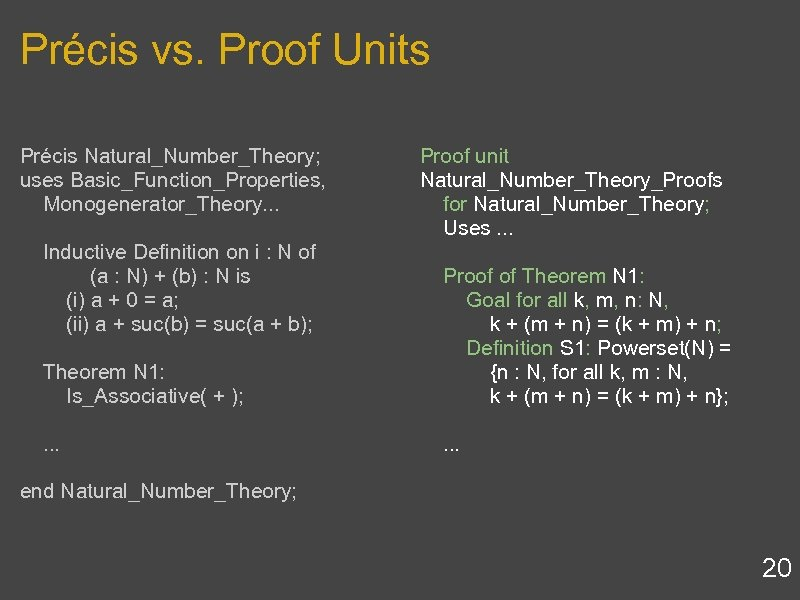Précis vs. Proof Units Précis Natural_Number_Theory; uses Basic_Function_Properties, Monogenerator_Theory. . . Inductive Definition on i : N of (a : N) + (b) : N is (i) a + 0 = a; (ii) a + suc(b) = suc(a + b); Theorem N 1: Is_Associative( + ); . . . Proof unit Natural_Number_Theory_Proofs for Natural_Number_Theory; Uses. . . Proof of Theorem N 1: Goal for all k, m, n: N, k + (m + n) = (k + m) + n; Definition S 1: Powerset(N) = {n : N, for all k, m : N, k + (m + n) = (k + m) + n}; . . . end Natural_Number_Theory; 20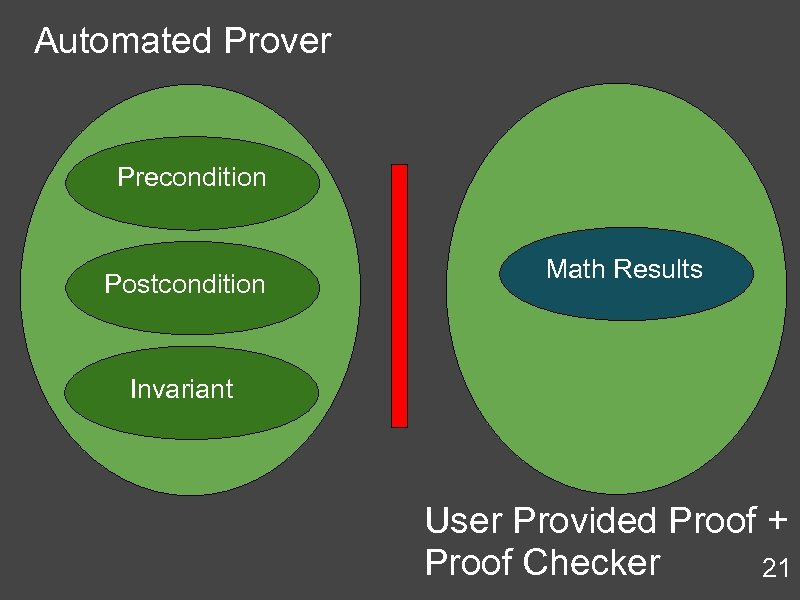Automated Prover Precondition Postcondition Math Results Invariant User Provided Proof + Proof Checker 21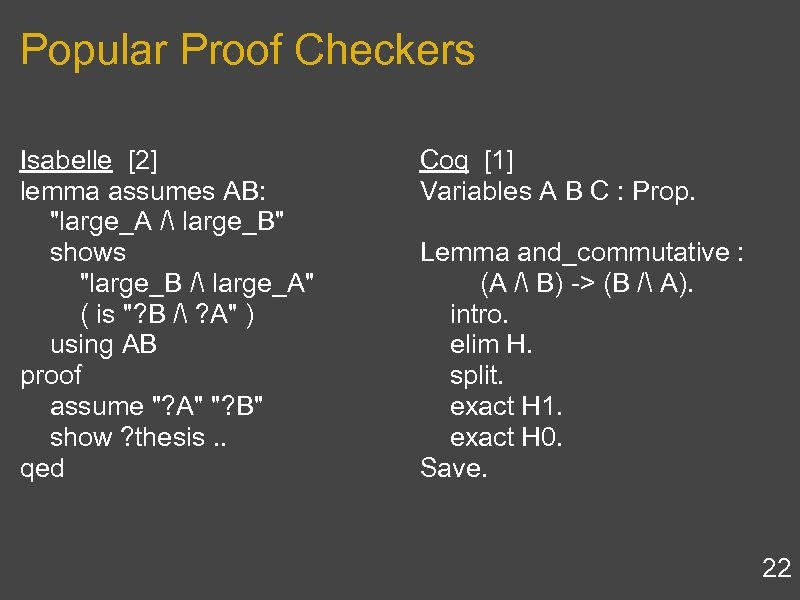Popular Proof Checkers Isabelle  lemma assumes AB: "large_A / large_B" shows "large_B / large_A" ( is "? B / ? A" ) using AB proof assume "? A" "? B" show ? thesis. . qed Coq  Variables A B C : Prop. Lemma and_commutative : (A / B) -> (B / A). intro. elim H. split. exact H 1. exact H 0. Save. 22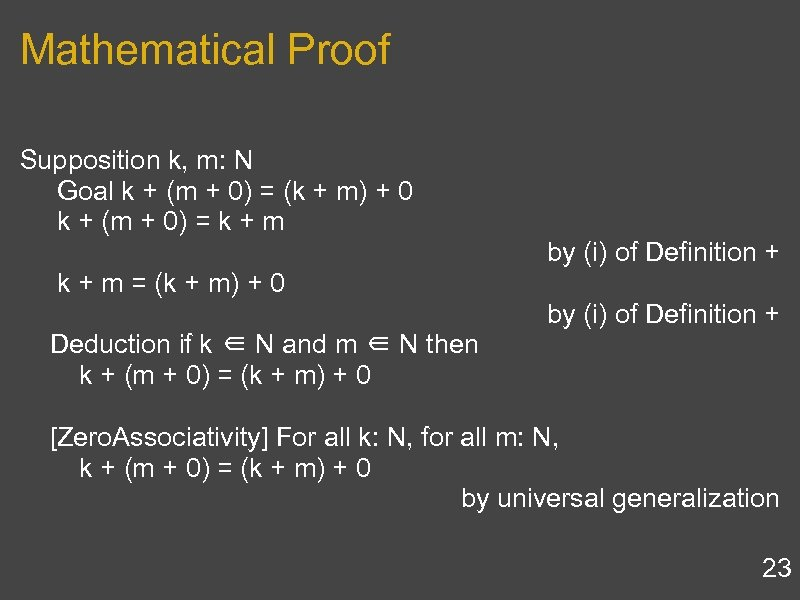Mathematical Proof Supposition k, m: N Goal k + (m + 0) = (k + m) + 0 k + (m + 0) = k + m by (i) of Definition + k + m = (k + m) + 0 by (i) of Definition + Deduction if k ∈ N and m ∈ N then k + (m + 0) = (k + m) + 0 [Zero. Associativity] For all k: N, for all m: N, k + (m + 0) = (k + m) + 0 by universal generalization 23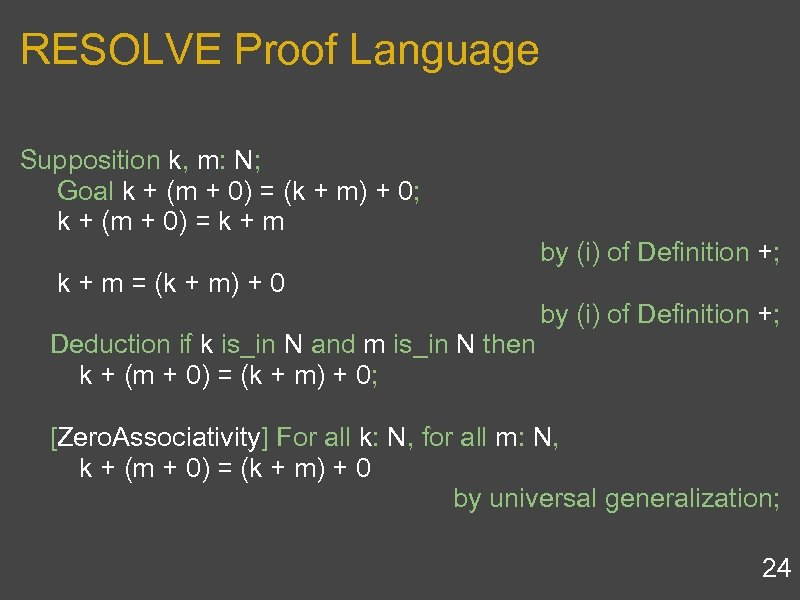RESOLVE Proof Language Supposition k, m: N; Goal k + (m + 0) = (k + m) + 0; k + (m + 0) = k + m by (i) of Definition +; k + m = (k + m) + 0 by (i) of Definition +; Deduction if k is_in N and m is_in N then k + (m + 0) = (k + m) + 0; [Zero. Associativity] For all k: N, for all m: N, k + (m + 0) = (k + m) + 0 by universal generalization; 24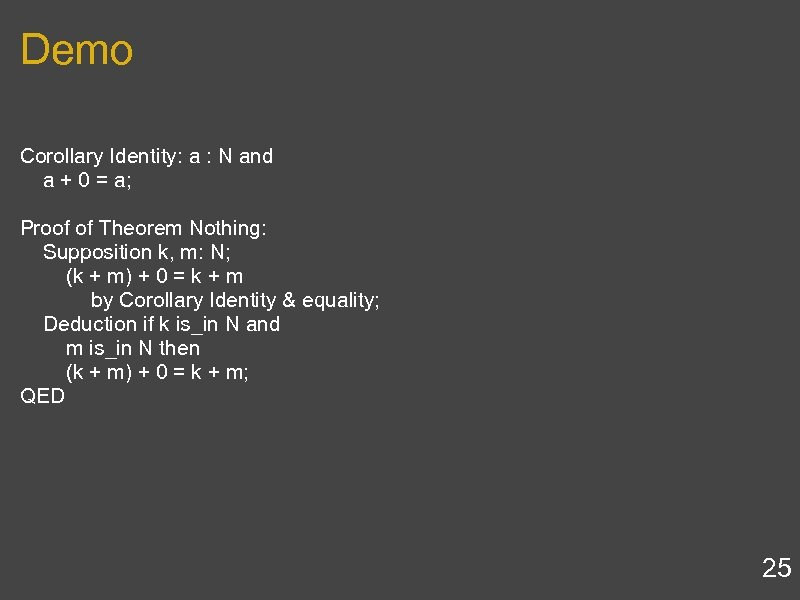Demo Corollary Identity: a : N and a + 0 = a; Proof of Theorem Nothing: Supposition k, m: N; (k + m) + 0 = k + m by Corollary Identity & equality; Deduction if k is_in N and m is_in N then (k + m) + 0 = k + m; QED 25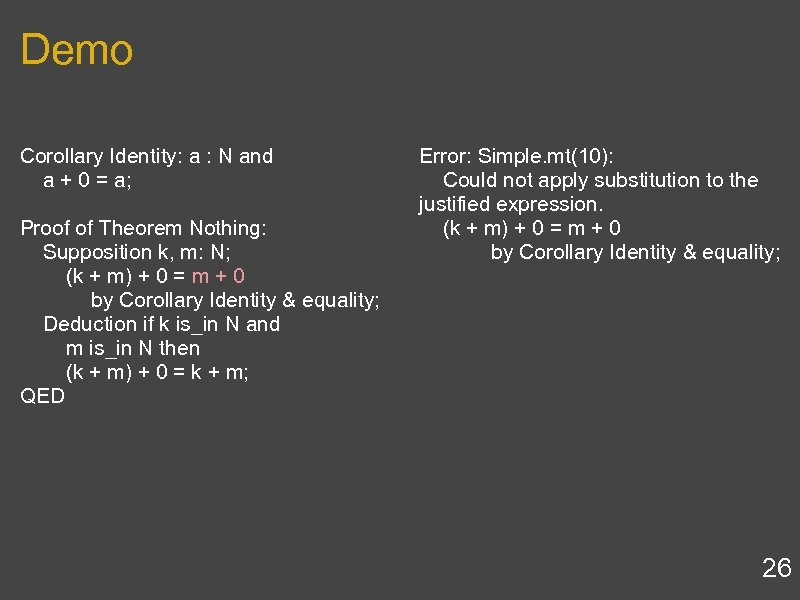Demo Corollary Identity: a : N and a + 0 = a; Proof of Theorem Nothing: Supposition k, m: N; (k + m) + 0 = m + 0 by Corollary Identity & equality; Deduction if k is_in N and m is_in N then (k + m) + 0 = k + m; QED Error: Simple. mt(10): Could not apply substitution to the justified expression. (k + m) + 0 = m + 0 by Corollary Identity & equality; 26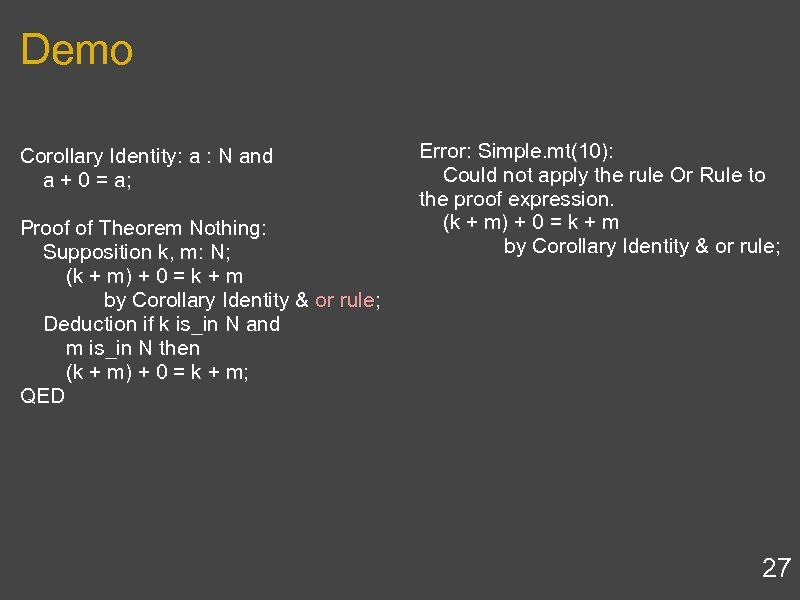Demo Corollary Identity: a : N and a + 0 = a; Proof of Theorem Nothing: Supposition k, m: N; (k + m) + 0 = k + m by Corollary Identity & or rule; Deduction if k is_in N and m is_in N then (k + m) + 0 = k + m; QED Error: Simple. mt(10): Could not apply the rule Or Rule to the proof expression. (k + m) + 0 = k + m by Corollary Identity & or rule; 27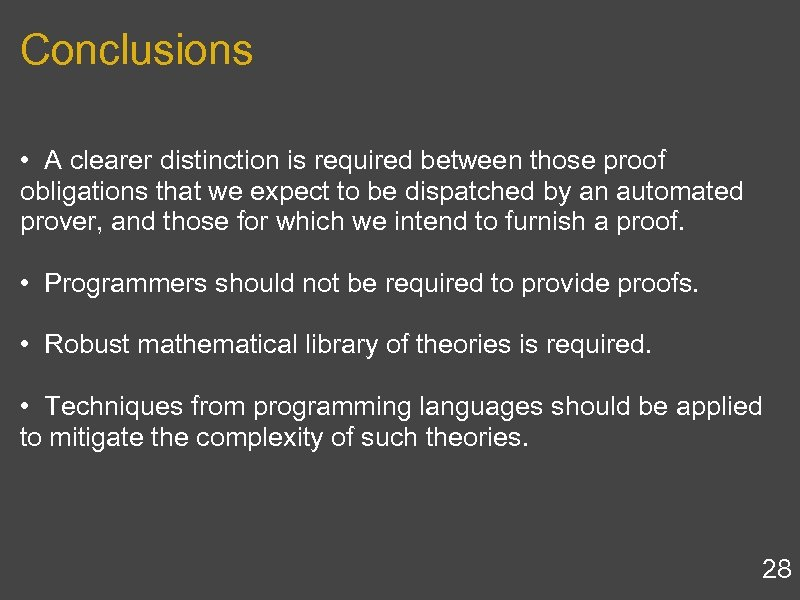Conclusions • A clearer distinction is required between those proof obligations that we expect to be dispatched by an automated prover, and those for which we intend to furnish a proof. • Programmers should not be required to provide proofs. • Robust mathematical library of theories is required. • Techniques from programming languages should be applied to mitigate the complexity of such theories. 28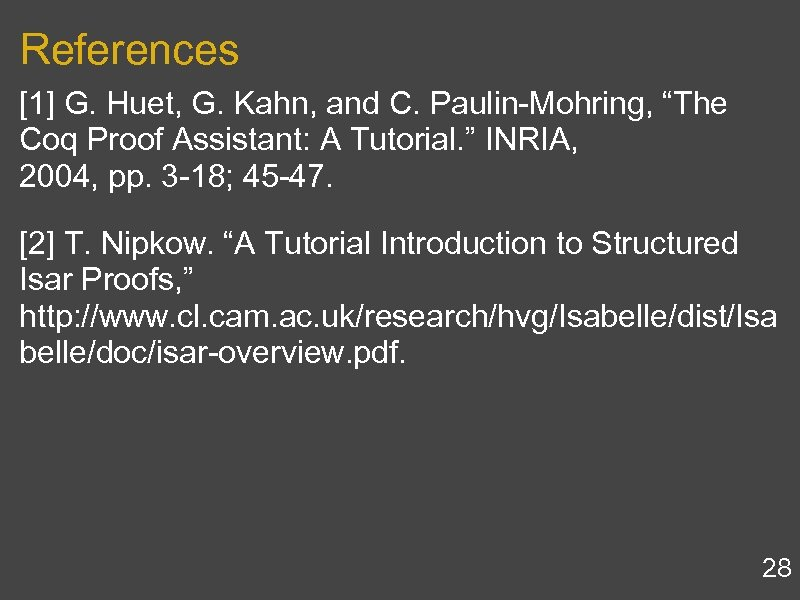References  G. Huet, G. Kahn, and C. Paulin-Mohring, “The Coq Proof Assistant: A Tutorial. ” INRIA, 2004, pp. 3 -18; 45 -47.  T. Nipkow. “A Tutorial Introduction to Structured Isar Proofs, ” http: //www. cl. cam. ac. uk/research/hvg/Isabelle/dist/Isa belle/doc/isar-overview. pdf. 28